# Twin Primes

The following is an attempt at probing the infinity of twin primes. I believe my approach and concepts are a little odd, but I was surprised by the remarkable numeric results for counting primes, as well as one of the terms I created after I cross-referenced it online. Please bear wih me.

The first thing I did was to draw a generic prime number, or the location of many different prime numbers in a single image.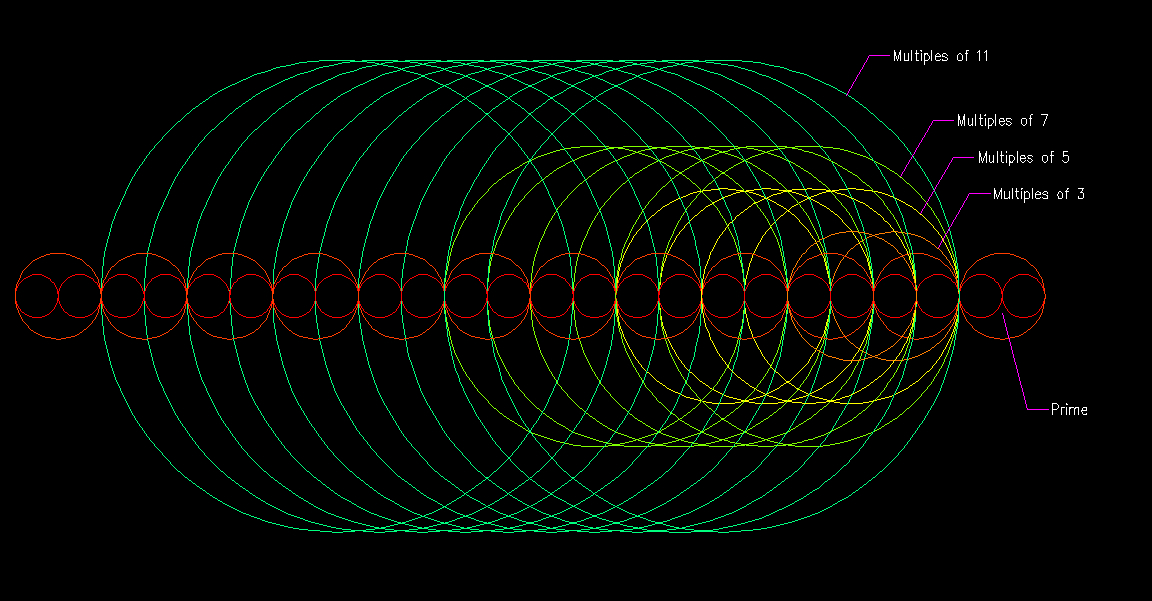This image simply tells us that for a prime number, multiples of the numbers in the sieve must fall in one of the locations shown. This is simply saying graphically that no multiple of any number bigger than "1" can fall on a prime number.

It can be discerned that the number "3" can fall on 2 locations adjacent to a prime number, the number "5" can fall on 4 locations adjacent to a prime number, the number "7" can fall on 6 locations, and so on. We can generalize this and state that a number "p" can fall on "p-1" locations adjacent to prime numbers. Pretty obvious stuff.

This is where it gets a bit odd. If we are sifting for prime numbers like on the "home" tab, then we could apply a probability to the map of factors for having a prime number at a certain point. For example, two thirds of multiples of 3 will not fall on the prime number above, because you can only have two locations out of the possible three locations where "3" can fall. In the same way, multiples of "5" can only fall on four possible locations, so this will happen four fifths of the time. We can apply this same procedure to the rest of the prime numbers included in the sieve, but in order to get a prime number, all these allowable locations for each circle in the sieve need to occur at the same time. This probability will be given by multiplying all the ratios: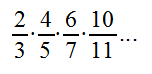This series can be expressed in the following manner: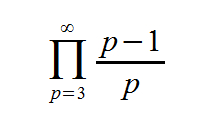This product series is the probability that a sieve will yield a prime number in a given point. That is up to the square of the largest prime number used in the sieve. This series converges to zero at infinity (because you never multiply by zero), so it basically says there is always a chance of finding a prime number as you use more and more terms in the product formula. It also suggests there are infinitely many prime numbers, which is a well known fact.

(Update): I've realized that I need to tell the formula to divide the total probability by 2. The reason is that the formula doesn't know it cannot apply this at multiples of "2", therefore we need to let it know it can only apply the total probability in half of all the locations!! so our corrected formula becomes:Remember, this formula will give you the probability of finding prime numbers up to the square of the largest prime number used in the sieve. I've input this into Excel and found that it is very close to Gauss' approximation of "n/log(n)", but it will overshoot after a while. I know why it overshoots, but I will post more on this later. It has to do with "die rolls" that are "not allowable".

After some thinking I've rewritten the formula so that it's more intuitive and easy to understand. The formula for counting primes is the following:Keep in mind that I brought the "1/2" into the product operator.

Now let's do the same thing with a generic location of twin primes: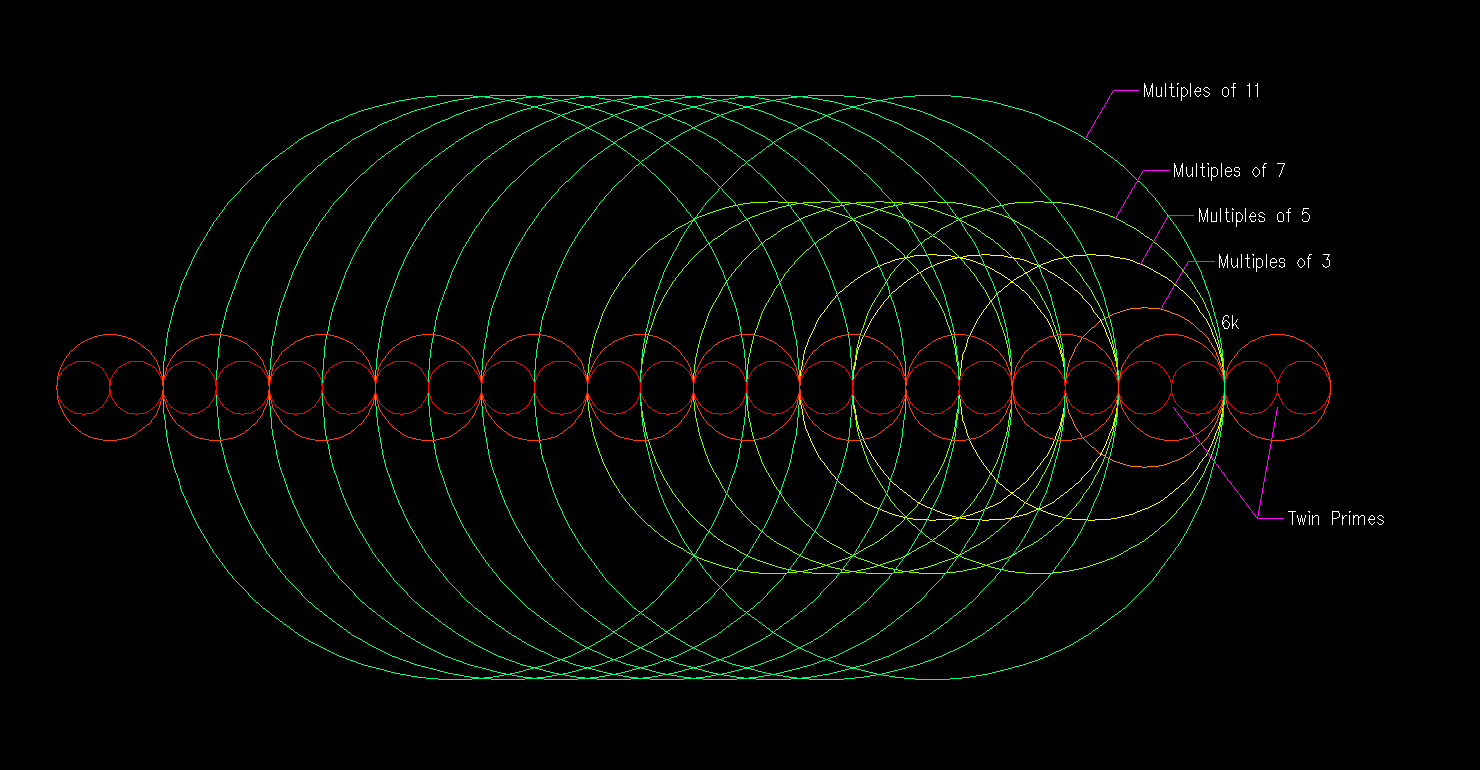This image shows that the number "3" can only fall on one location, the number "5" on three locations, the number "7" on five locations, the number "11" on 9 locations, and so on. So a number "p" can fall on "p-2" locations.

Dividing "p-2" over "p" should represent the probability that a certain prime used in the sieve will yield a twin prime location, so if we apply a similar product of all the terms, we get:(Update): Using a similar insight as in the previous update, we now have 3 locations defined. The first is the twin prime at "6k-1", the second is the location "6k", and the third is the location at "6k+1", but we want this to occur at only one of the 3 possible defined locations, therefore I believe we need to divide by "3" instead of "2" this time. I think this is correct especially after running the numbers.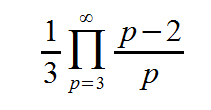This series converges to zero faster than the first one as you input more and more terms, but it doesn't ever reach zero either. This is the probability of finding a twin prime location, so if it never really reaches zero then there is always a chance of yielding twin primes with a sieve. Again, this series will overshoot the amount of primes, and again it's because of "die rolls" that are "not allowed", but it should always converge to zero at infinity as long as there are "die rolls" that are "allowable". Thus, I tend to believe that there should be an infinite number of twin primes...

Again, the following expression counts twin primes: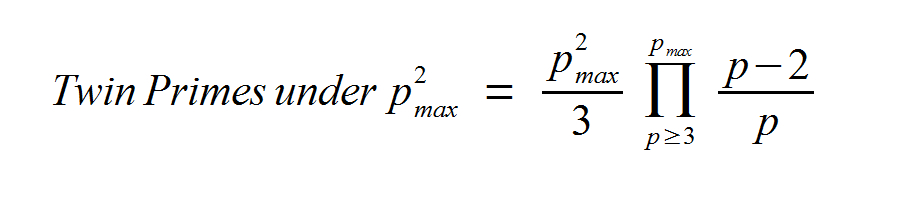Notice that the "1/3" is not brought into the product operator.

It also turns out that this formula applies perfectly well for "Cousin Primes" (Prime Numbers separated by 4 instead of 2). More on this later.

Why do these formulas overshoot? Ok, here's where the nightmare begins. It turns out that we are including all possible circle combinations, the problem arises when a combination of circles suggests a number higher than the square of the largest number used to build the sieve. For example, if all circles fall on the same location and still allow for the twin primes to occur, then that combination is not allowable, because it is beyond the "range of the sieve". So we need to see what ratio of the combinations suggest numbers under the square of the largest prime used to build our sieve. If you watch the videos in the "Video Summary" I have done some work on this, but I will post more when I have more insights.

I can tell you that the excel spreadsheets I have to probe for these "allowable combinations" are the nightmare from hell !! They tap into the limits of my computing power! So, I need to come up with some more advanced programming methods for this.

I also created a term which represents the probability that, given there already is a prime, a sieve will yield another prime 2 units apart . Since I'm assuming that there is already a prime number in the first place, then that probability still needs to enter the equation also, so the next term is incomplete as you'll see further down. The formula for this term is the following:Now, I applied the concept of "conditional probability". Assuming you already have a prime number, what is the probability that there will be another prime number at p+2 or p-2? To do this, you have to divide the probability of having twin primes by the probability of having a single prime in the first place. The formula is as follows: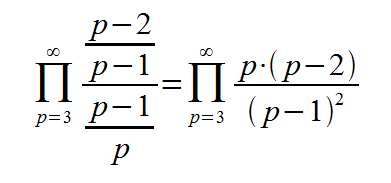Incredibly this term already exists, I believe Hardy and Littlewood were the ones who introduced it, although I could be mistaken. I have no idea how they came up with this constant, I would doubt they used the same thought process as me, simply because I'm just an amateur. Or perhaps this "conditional probability" is an accidentally crazy idea.

I was reluctant that this infinite product (using only primes) would actually converge, so I downloaded an excel spreadsheet from the internet with over 65000 prime numbers in a column, and I introduced this formula to each prime number in the list. It seems that when you multiply all the terms, the series converges to "0.6601618...etc" which was a relief. When I realized this term already existed, I was actually quite happy.

This is another update, but I would think that this "ratio" needs to be evaluated among pairs of primes. First, we need to account for the probability of having 2 primes, and then the probability that the 2 primes are twins (as in the conditional probability above). So we need to use this ratio in the distribution of "pairs of primes". Maybe I'll have more on this later.

Make a Free Website with Yola.